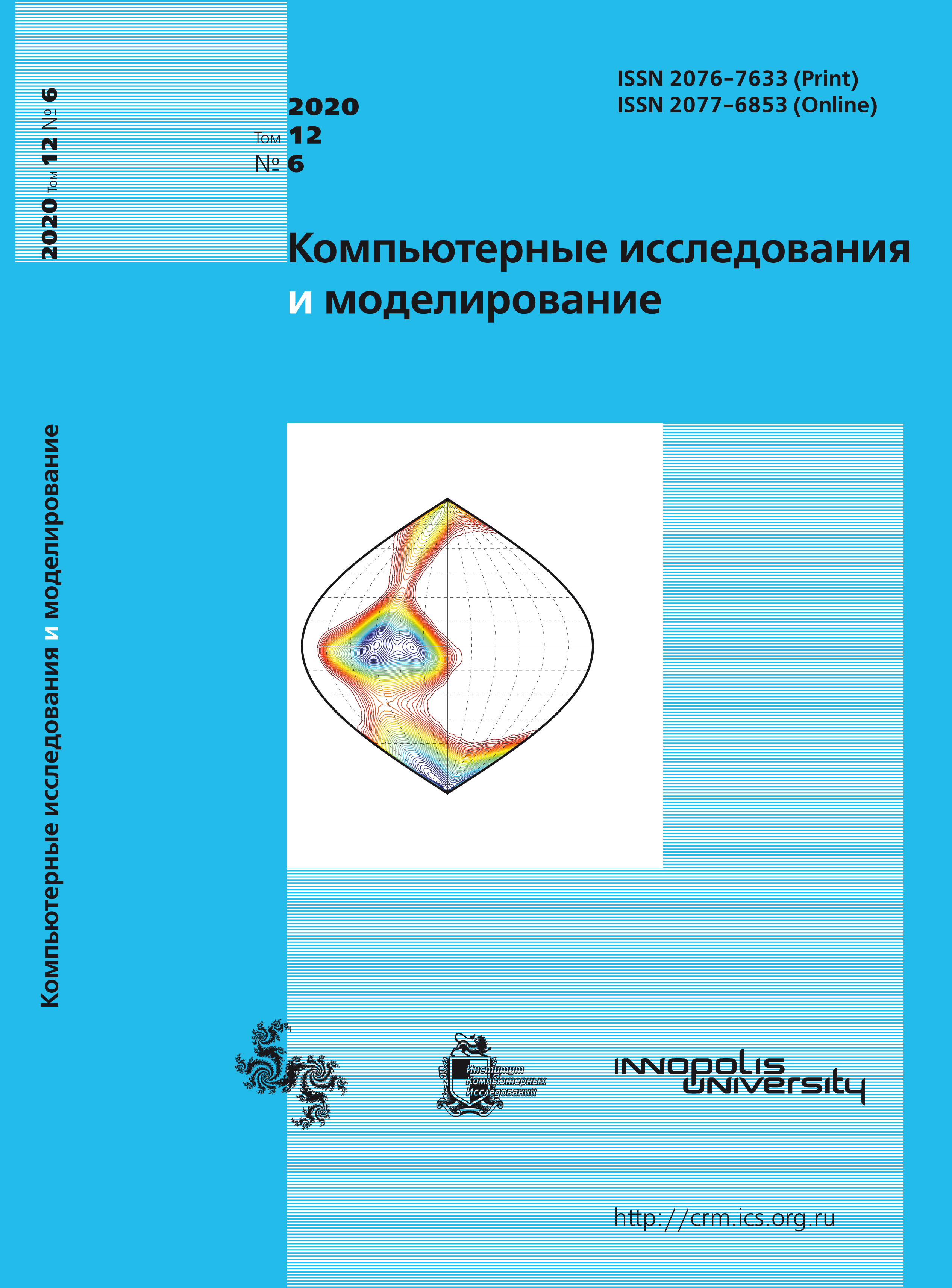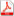Issue 6, 2020 Vol. 12

# All issues

The method of numerical solution of the one stationary hydrodynamics problem in convective form in $L$-shaped domain

" />

The method of numerical solution of the one stationary hydrodynamics problem in convective form in $L$-shaped domainpdf (3678K)

An essential class of problems describes physical processes occurring in non-convex domains containing a corner greater than 180 degrees on the boundary. The solution in a neighborhood of a corner is singular and its finding using classical approaches entails a loss of accuracy. In the paper, we consider stationary, linearized by Picard’s iterations, Navier – Stokes equations governing the flow of a incompressible viscous fluid in the convection form in $L$-shaped domain. An $R_\nu$-generalized solution of the problem in special sets of weighted spaces is defined. A special finite element method to find an approximate $R_\nu$-generalized solution is constructed. Firstly, functions of the finite element spaces satisfy the law of conservation of mass in the strong sense, i.e. at the grid nodes. For this purpose, Scott – Vogelius element pair is used. The fulfillment of the condition of mass conservation leads to the finding more accurate, from a physical point of view, solution. Secondly, basis functions of the finite element spaces are supplemented by weight functions. The degree of the weight function, as well as the parameter $\nu$ in the definition of an $R_\nu$-generalized solution, and a radius of a neighborhood of the singularity point are free parameters of the method. A specially selected combination of them leads to an increase almost twice in the order of convergence rate of an approximate solution to the exact one in relation to the classical approaches. The convergence rate reaches the first order by the grid step in the norms of Sobolev weight spaces. Thus, numerically shown that the convergence rate does not depend on the corner value.

Keywords: hydrodynamic problem with singularity, weighted finite element problem
Citation in English: Rukavishnikov V.A., Rukavishnikov A.V.

The method of numerical solution of the one stationary hydrodynamics problem in convective form in $L$-shaped domain // Computer Research and Modeling, 2020, vol. 12, no. 6, pp. 1291-1306

Citation in English: Rukavishnikov V.A., Rukavishnikov A.V.

The method of numerical solution of the one stationary hydrodynamics problem in convective form in $L$-shaped domain // Computer Research and Modeling, 2020, vol. 12, no. 6, pp. 1291-1306

DOI: 10.20537/2076-7633-2020-12-6-1291-1306

Full-text version of the journal is also available on the web site of the scientific electronic library eLIBRARY.RU

The journal is included in the Russian Science Citation Index

The journal is included in the List of Russian peer-reviewed journals publishing the main research results of PhD and doctoral dissertations.

International Interdisciplinary Conference "Mathematics. Computing. Education"

The journal is included in the RSCI

Indexed in Scopus